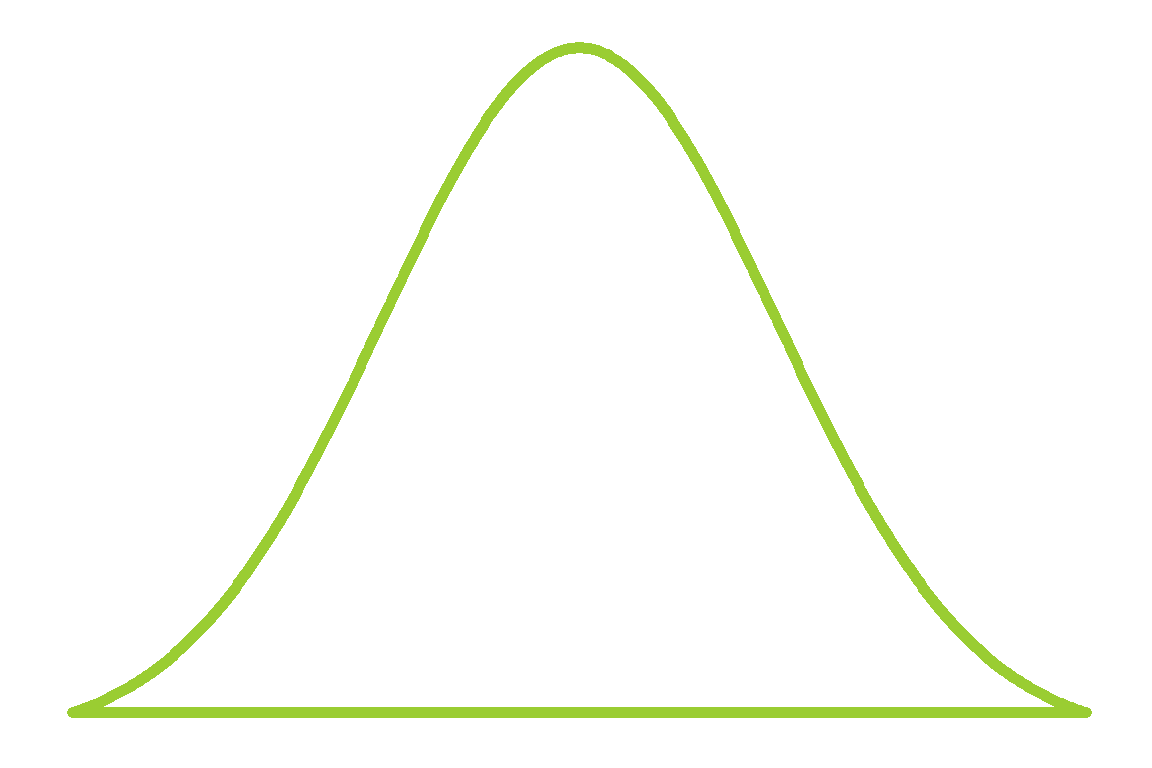When I was in school I was taught that if you want to see if a data set is normally distributed, you can create a graph called a QQ plot. If the points on the scatter plot make a straight line, the data is normally distributed. I’m a bit embarrassed to say this but at that at the time, I only had a vague understanding of why this works and just accepted it as some dark sorcery. Much later, I came up with a different way of phrasing the concept that made it clear to me. My intention with this essay is to give a long winded explanation of this idea in a way most people, statistically literate or not, can understand.

The motivating question is ‘how can we get a feel for whether a data set resembles a theoretical distribution?’ Many statistical techniques start with the phrase ‘assume the data is normally distributed.’ This essay will give you a technique for assessing that assumption. We will explore questions about shapes of data and learn how QQ plots help in this dilemma.

I’ve gotten my dirty mitts on the prices of avocados from this kaggle page. We’ll see if the prices look roughly normal or whether they fit a different distribution better.

You can see the code used to generate this document here.

### Distributions

In statistics, we often talk about a population which is the set of all possible measurements and samples which are subsets of the population. For our example, we have a sample of organic avocado prices from 2016 & 2017 from the Haas corporation. The population we are sampling from could be the set of all avocado prices in the U.S. from 2016 to 2017.

We can use something called a theoretical distribution to describe the shape that a population takes mathematically. It’s easy to think of these as curves. By using a distribution, we unlock a lot of fancy algebra that supports most of the statistical techniques you’ve heard of.

Here’s an example of everyone’s favorite theoretical distribution, the standard normal: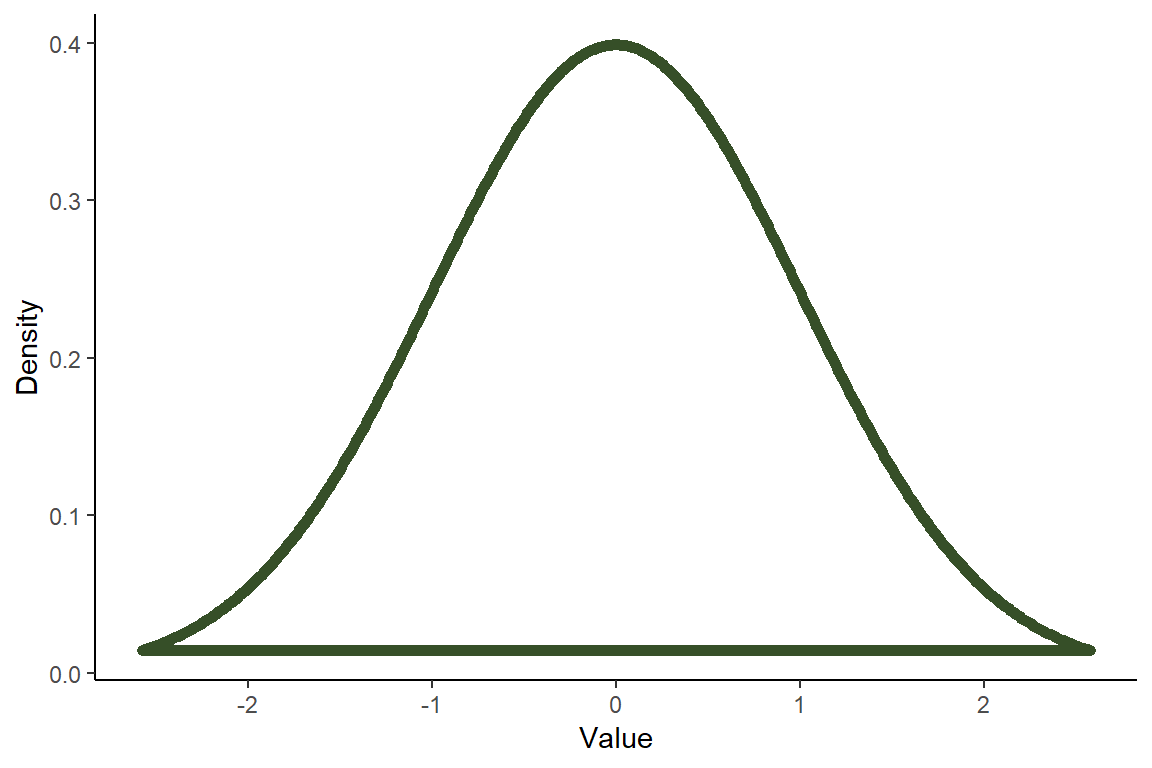Values near the center are much more likely to occur than values farther out in either direction because the distribution is more dense (taller). Now let’s take a look at the 5,668 avocado prices in the sample data set. Here is the scourge of millennial finances across the nation: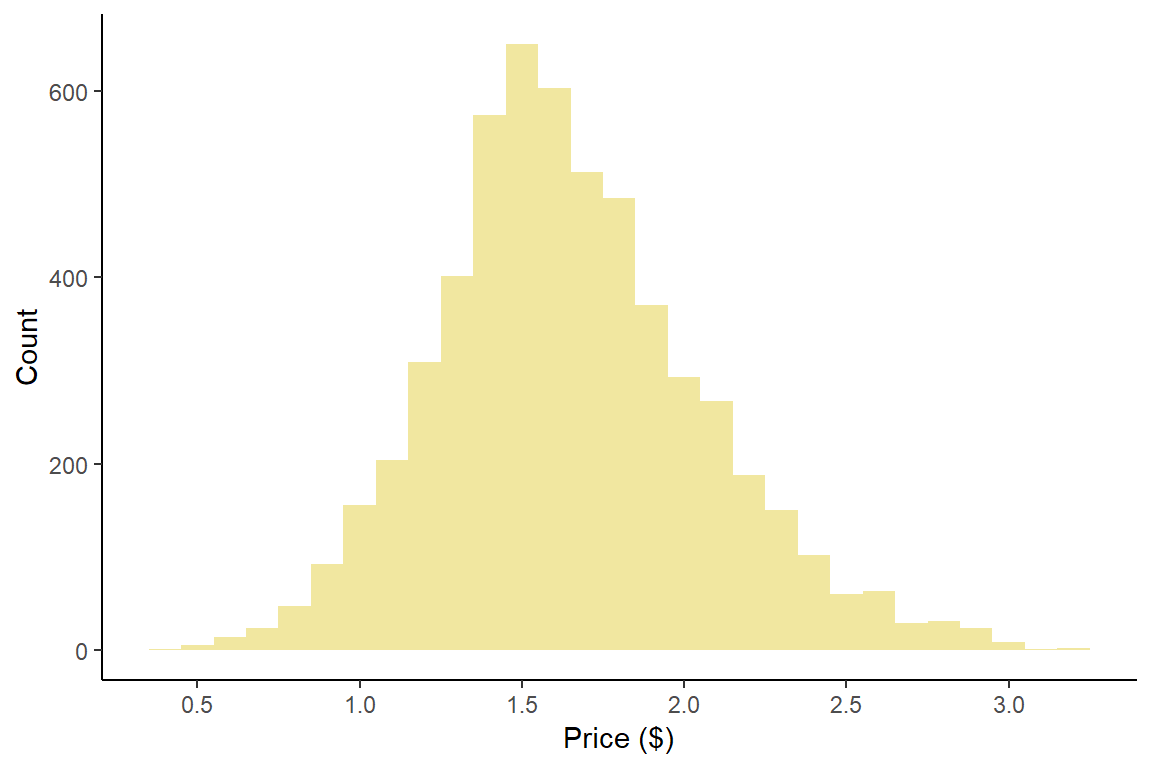The histogram shows us that most avocados cost between $1.00 and$2.50. The sample has a single peak and is pretty symmetrical so we could easily believe that this data comes from a normal distribution. If we put a normal curve on the graph, it fits loosely like the skin of a ripe avocado.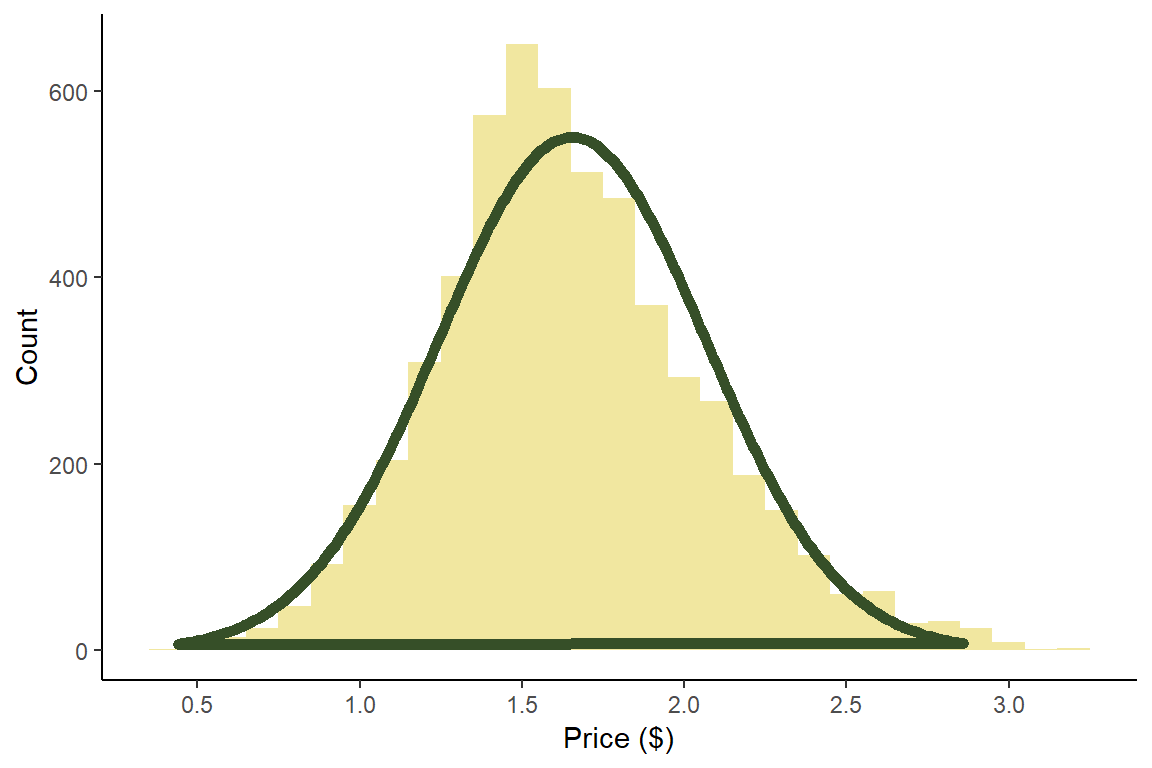While the curve fits this data set well, this is often not the case. Many statistical techniques require the data to look roughly like one distribution or another. Staring at the histogram is one way to assess this. Another is to consider the quantiles.

### Quantiles

Quantiles are locations on distributions that are larger than a specific amount of the data. These are commonly reported for standardized tests and child development tables. For example, a 36 month old girl weighing 28 lbs. (13 kg) is at the 25$$^{th}$$ weight quantile. This means that she is heavier than 25% of the girls her age and lighter than 75%.

Visually, the N$$^{th}$$ quantile can be represented as locations on a distribution for which N% of the area under the curve is to its left. Here is the standard normal distribution with the 40th quantile marked. The 40th quantile is -0.253. You’ll notice that this is a little bit to the left of the center because the 50th quantile (median) is the exact center. The tan area is 40% of the total area.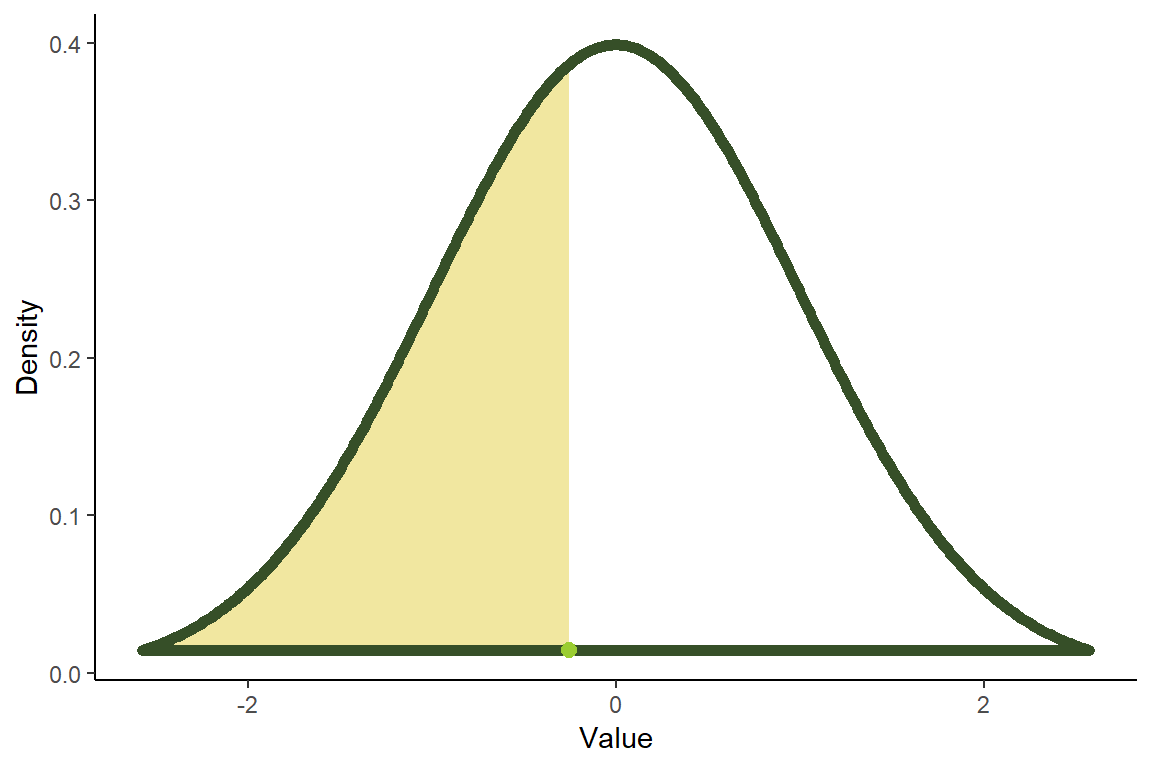If we mark many quantiles on the distribution, we can see that there is a pattern of sorts in their spacing. Below are the 5th, 10th, 15th… quantiles. Each represents an increase of 5% of the total area.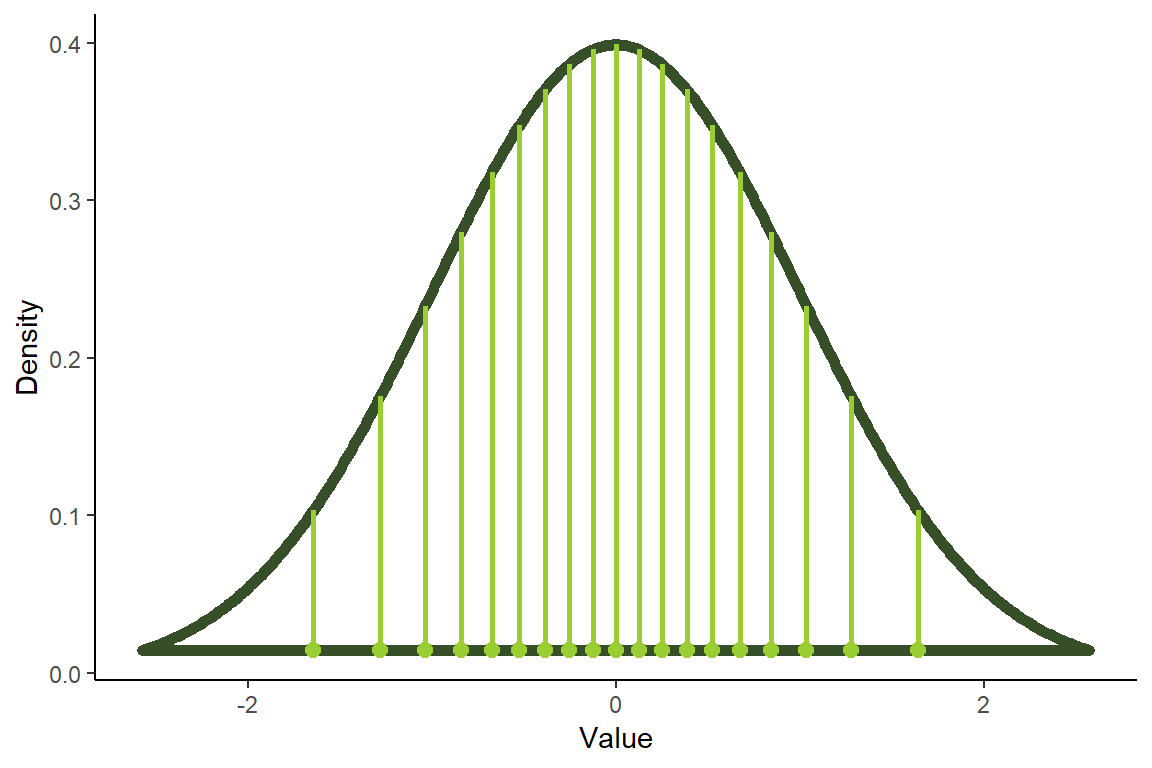Because each slice of the distribution is 5% of the total area and the height of the graph is changing, the slices have different widths. It’s like we’re trying to cut a strange shaped cake into 20 equal pieces using parallel cuts. The slices at the center must be thinner since the distribution is denser (taller) than on the edges. If we just look at the points by themselves, we get a pretty pattern: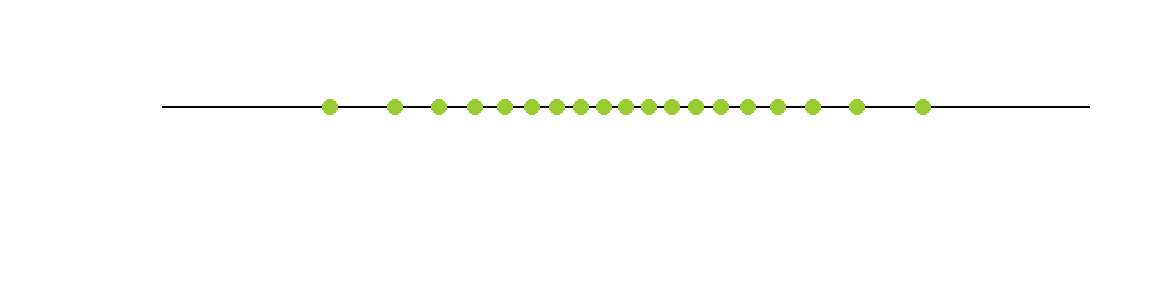This method of viewing the quantiles of a distribution is what made QQ plots clearer to me. The sequence of differently spaced dots allows us to see a two dimensional curve as a one dimensional string of points. The spaces between the points describe the relative height of the curve. Smaller spaces indicate that the curve is taller while larger gaps indicate a less dense portion of the distribution.

I like to think of this pattern of points as the distribution’s signature. It’s a way of characterizing the distribution in a code of sorts. You might imagine that if we drew the signature with 100 points, instead of 19, that a computer could roughly recreate the distribution by comparing the relative sizes of the gaps between points.

Let’s compare the normal distribution’s signature to a related distribution: a chi-squared distribution $$(\chi^{2}(2))$$. Don’t worry too much about the name or fancy symbol. It’s just another type of distribution. This is what it looks like with successive 5% quantiles: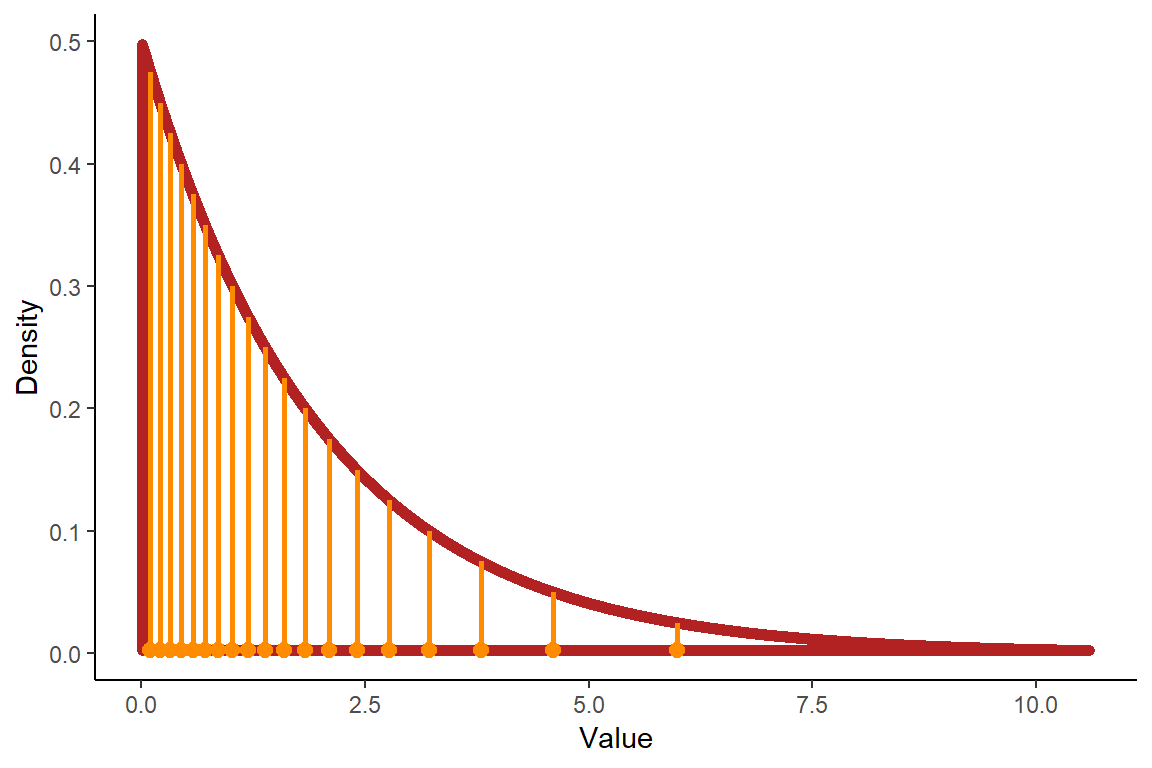Here is chi-squared’s signature:Notice how the symmetry (or lack thereof) of each distribution affects their signatures. The normal distribution’s quantiles are mirrored on the left and right of the 50th percentile (median). The chi-squared is very dense on the left hand side and then the spacing between the points grows as you move to the right.

Take a look at the distributions below. Try and envision what their signatures will look like and then click the tabs below to see them.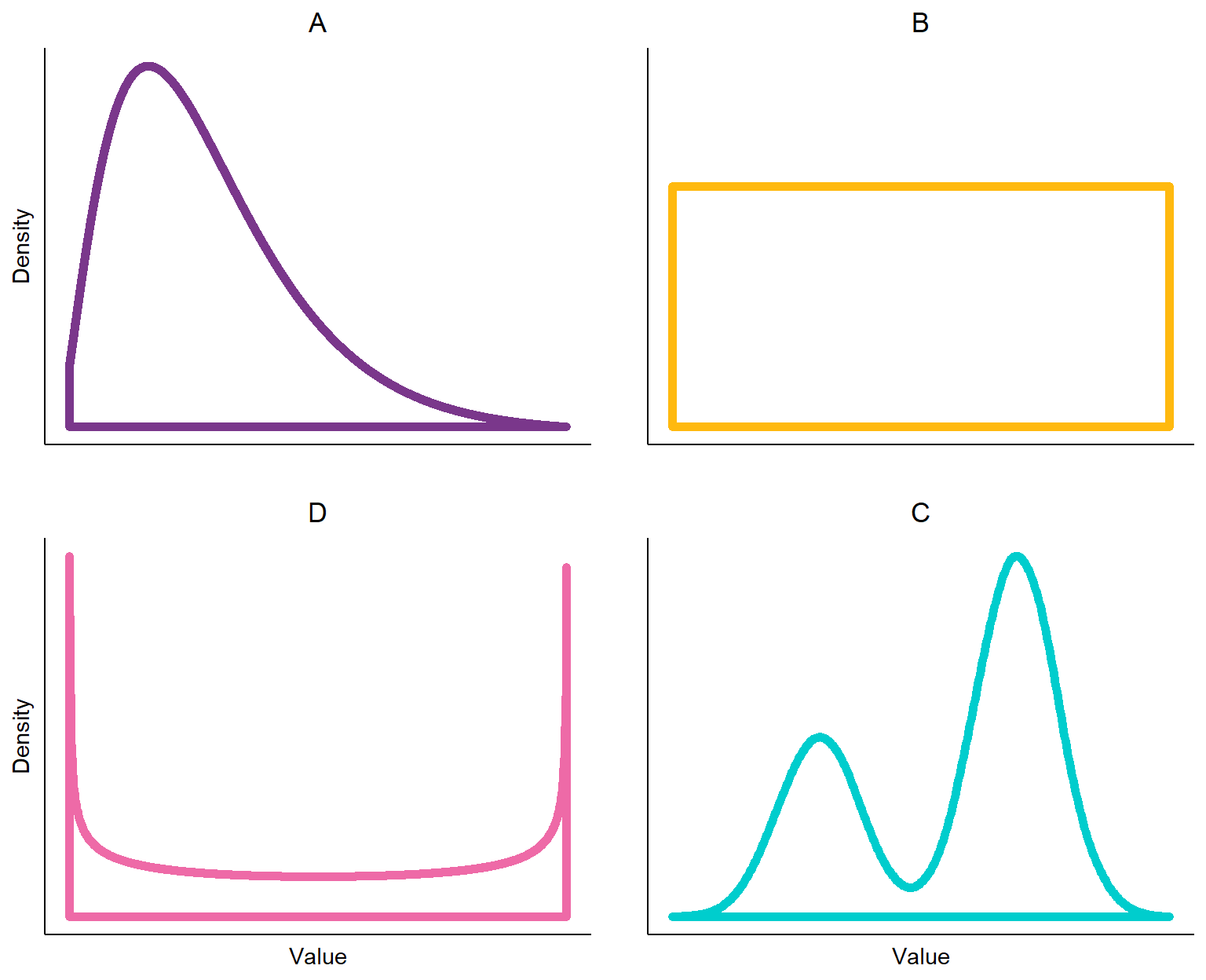#### Solutions

##### A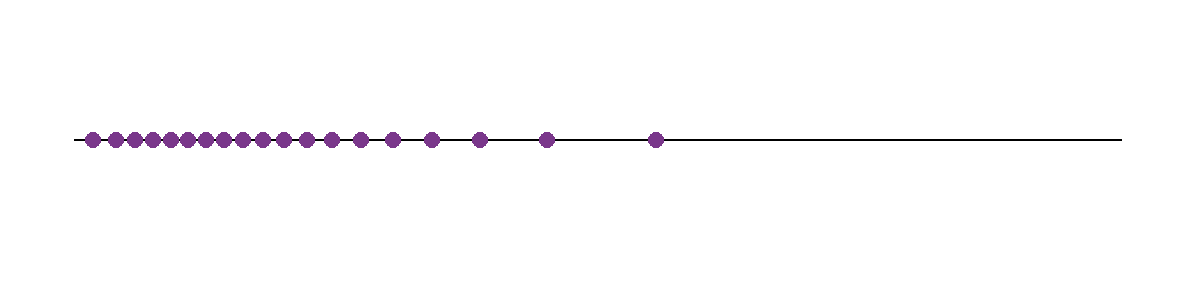The purple graph $$(\chi^{2}(4))$$ is a cousin of the chi-squared distribution we looked at before. Like the normal distribution, it has a single peak but it’s not symmetrical. Notice how the signature trails off on the right side as the distribution becomes less dense.

##### B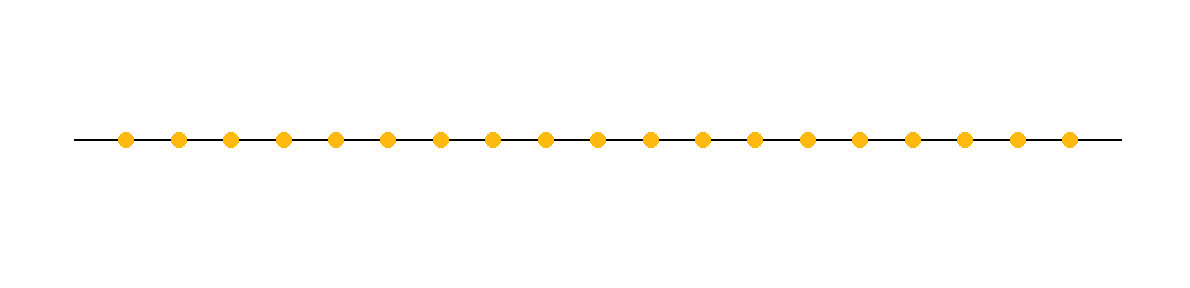This is the uniform distribution; each value has the same probability. Cutting this distribution into 20 slices requires 19 identically spaced cuts.

##### C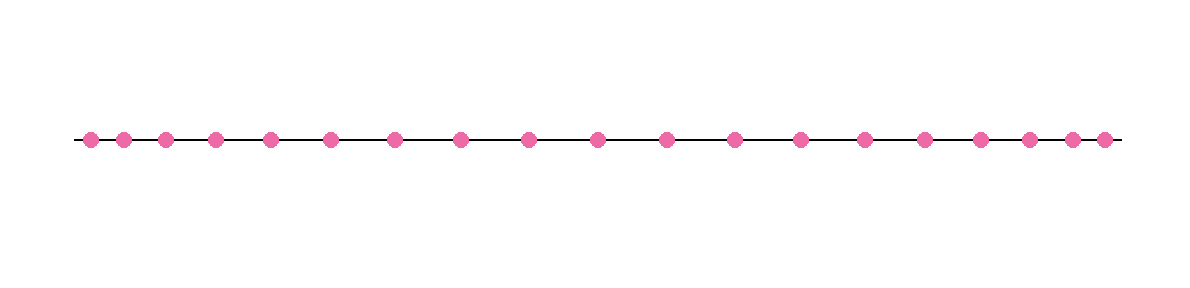Here we have a version of the beta distribution. Its signature looks a lot like the uniform distribution but the little horns on the sides warp the signature away from the center.

##### D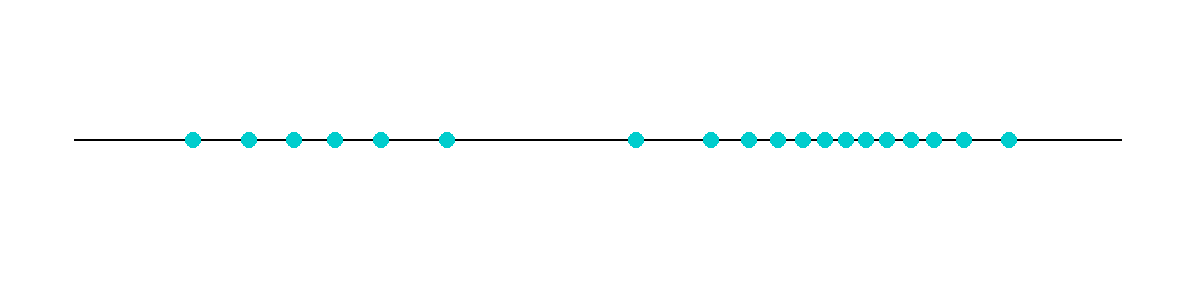This is a distribution I simulated. I took two normal distributions with different means and sampled them at a 1:2 ratio. The signature displays the two different peaks as clusters of points and the valley as a large gap in the middle.

### QQ Plots

Let’s return to the original question: how can we get a feel for how similar or different two distributions are?

Quantile-quantile (QQ) plots are one way of accomplishing this. They are formed by lining up the ordered quantiles of two distributions or samples as the vertical and horizontal axes of a graph. We are essentially comparing the signatures of two distributions by looking at the spacing between the quantiles. Let’s start with a bit of a silly example where we plot a standard normal distribution against itself. Its signature looks like this: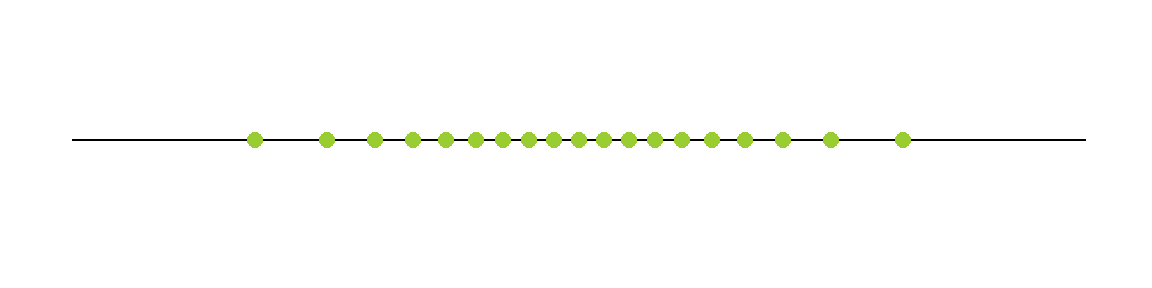Here is the normal distribution’s signature plotted against itself: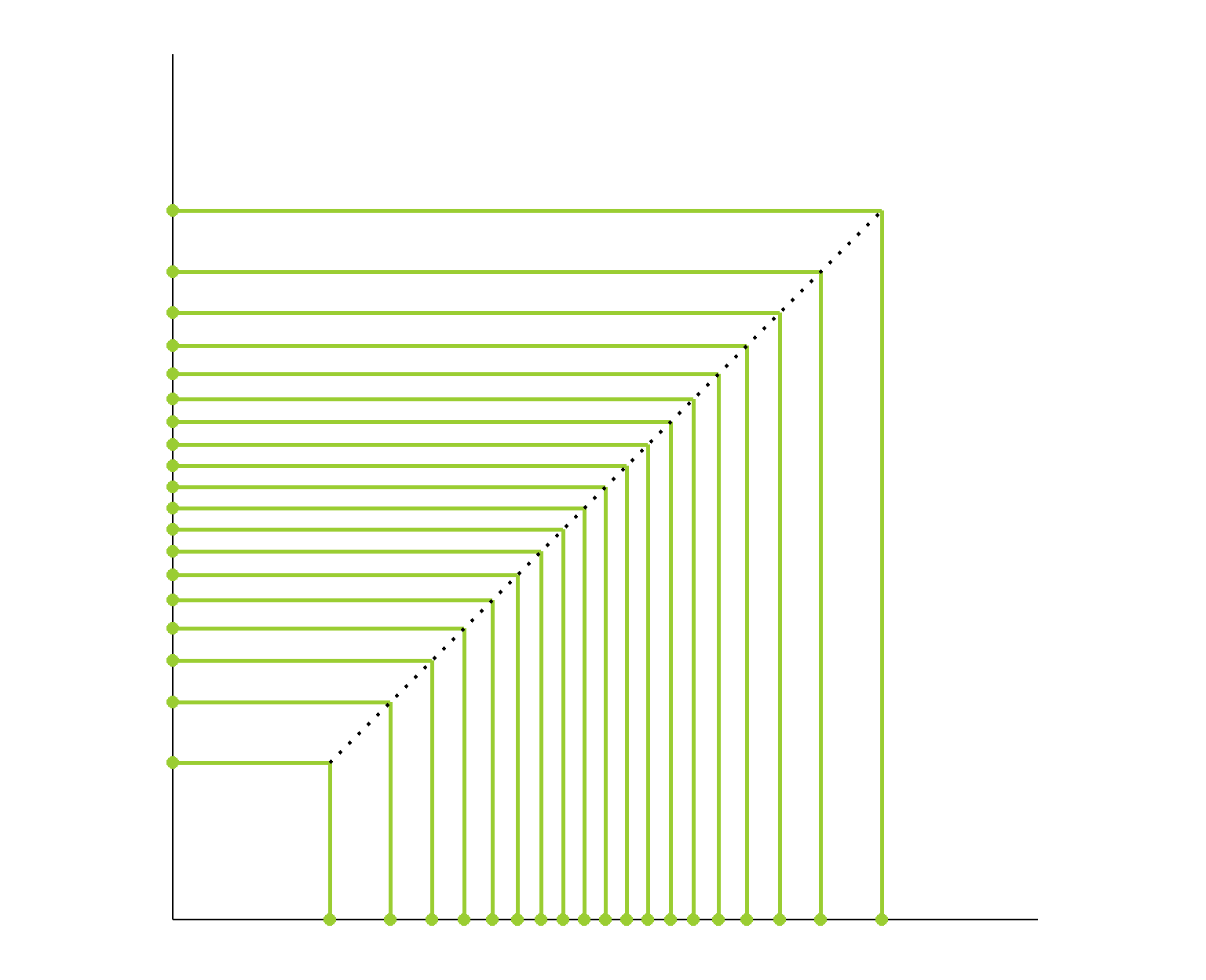This diagram shows us that the spacing between the quantiles is the same between the signatures. As we move from left to right on the horizontal axis, the size of the gap between the points changes. The corresponding quantiles on the vertical axis change by the same amount so all of the rectangles have the same ratio of length to width. This allows us to draw a straight line through the upper right corners of the rectangles.

Now let’s compare the signatures of the normal distribution and the chi-square(4) distribution that we just looked at. Each has a single peak but the chi-squared is not as symmetric: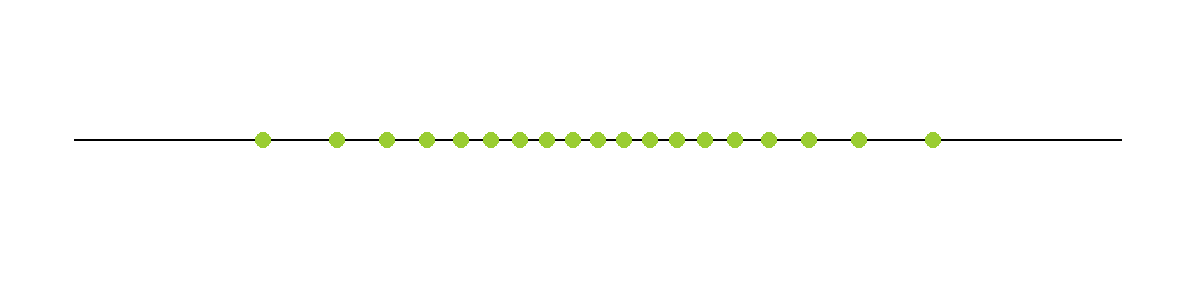Here is the plot of matching the quantiles of the chi-squared(4) and normal distributions. I’ve again plotted these quantiles over 98% of each distribution’s range. The chi-squared distribution is skewed so its quantiles are packed into a smaller portion of its axis.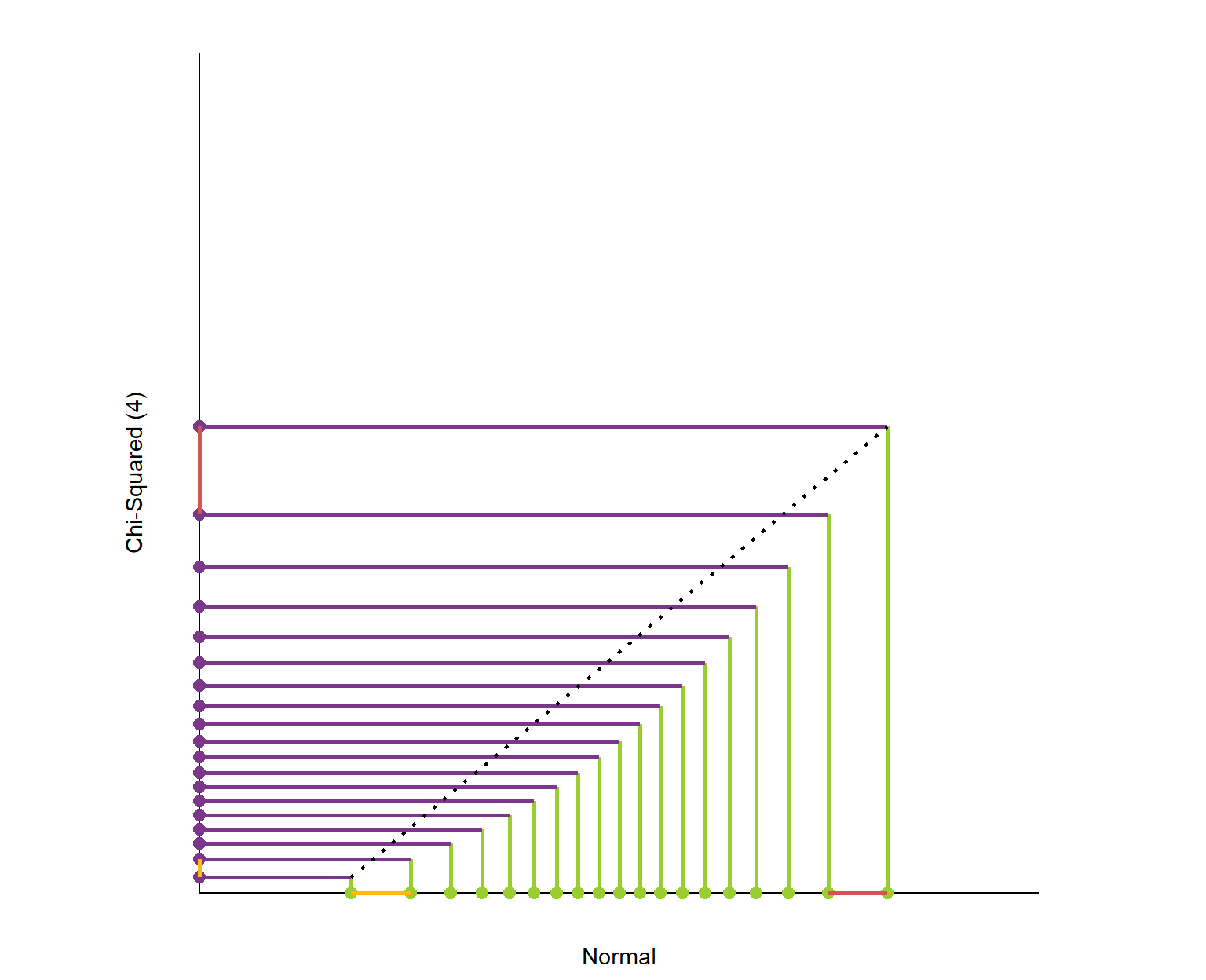What is this graph telling us? It shows that the exchange rate between the quantiles of the two distributions is not constant.

I’ve highlighted a comparison here with yellow and red. Because the normal distribution is symmetric, the red and yellow segments along the horizontal axis are the same length. By comparison, the corresponding segments on the chi-square’s axis are wildly different. This fluctuating exchange rate is what is causing the curved shape in the intersections of the two distributions quantiles. On the left hand side of the graph, the gaps between quantiles are larger for the normal distribution. By the time we reach the right hand side, the gaps are larger for the chi-squared.

This is the essence of a QQ plot. We’re curious if the signatures of the two distributions are similar. Do they have a similar sequence of spaces between their quantiles? If we’re thinking about signatures as encoded versions of distributions, similar signatures should indicate similar distributions.

Note that with these plots we’re not really interested in the scales of the graphs; if we multiplied all the quantiles of a distribution by 100, its signature will look the same. What’s important to determining if two distributions are similar is that the exchange rate between the quantiles is constant. Is a large gap in one distribution mirrored with a large gap in the other?

Now that we’ve taken a tour of the composition of a QQ plot, let’s return to our original question. We had this histogram of avocado prices and were curious how well it matched up with the theoretical normal distribution with the same mean and standard deviation:I’ve computed a few sample quantiles (every 10%) from the avocado data and lined them up with the corresponding quantiles of a normal distribution with the same mean and standard deviation as our sample. I’ve also calculated slopes to the next quantile so we can see how constant it feels:

Quantile Sample ($) Theoretical ($) Slope to Next Point
10% 1.16 1.13 1.06
20% 1.33 1.31 1.18
30% 1.44 1.44 1.38
40% 1.52 1.55 1.11
50% 1.61 1.65 1.00
60% 1.72 1.76 1.00
70% 1.83 1.87 0.87
80% 1.98 2.00 0.82
90% 2.20 2.18

You can see that for each quantile, the prices are very similar and the slope doesn’t vary a whole lot. The QQ plot will be formed by lining up the signatures of the sample (tan) and theoretical normal (green). Here are the signatures using 5% quantiles: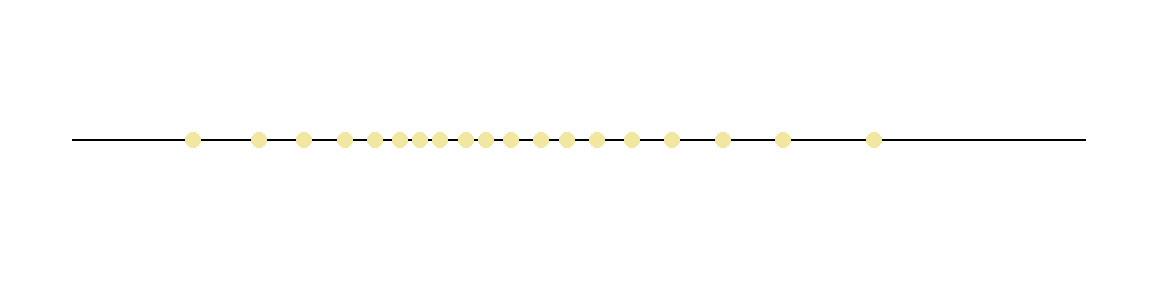Here is the QQ plot with the sample’s signature as the x coordinates and the normal’s signature as y: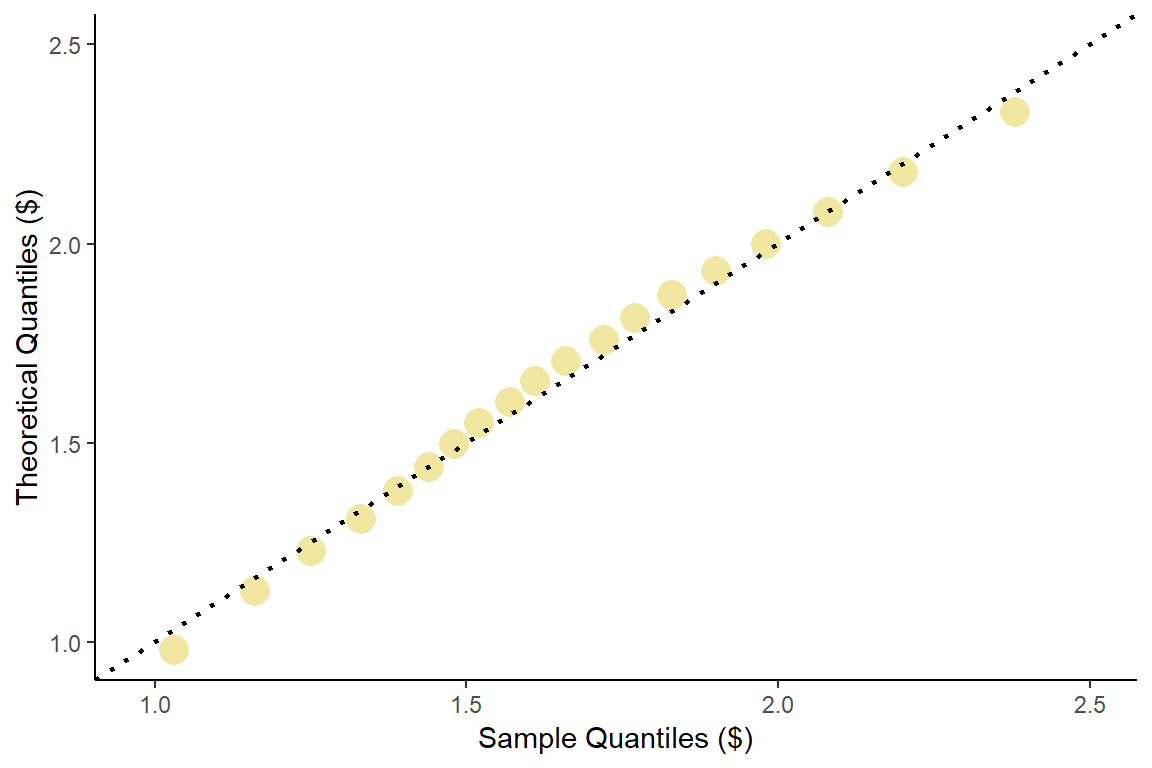The nineteen quantiles plotted above hug the reference line quite well and this gives further evidence that the sample of avocado prices we have resembles a normal distribution. Notice how on the signatures, the sample’s first and last quantiles are a bit larger than the corresponding points on theoretical normal. This is borne out on the QQ plot in that the first and last points are slightly below the reference line.

There are a number of different flavors of these plots. Many software applications compute a quantile for each data point you have. For example, a data set with 50 observation will be plotted against 2% quantiles because 50*2 = 100. Here’s our entire data set plotted against their corresponding theoretical quantiles: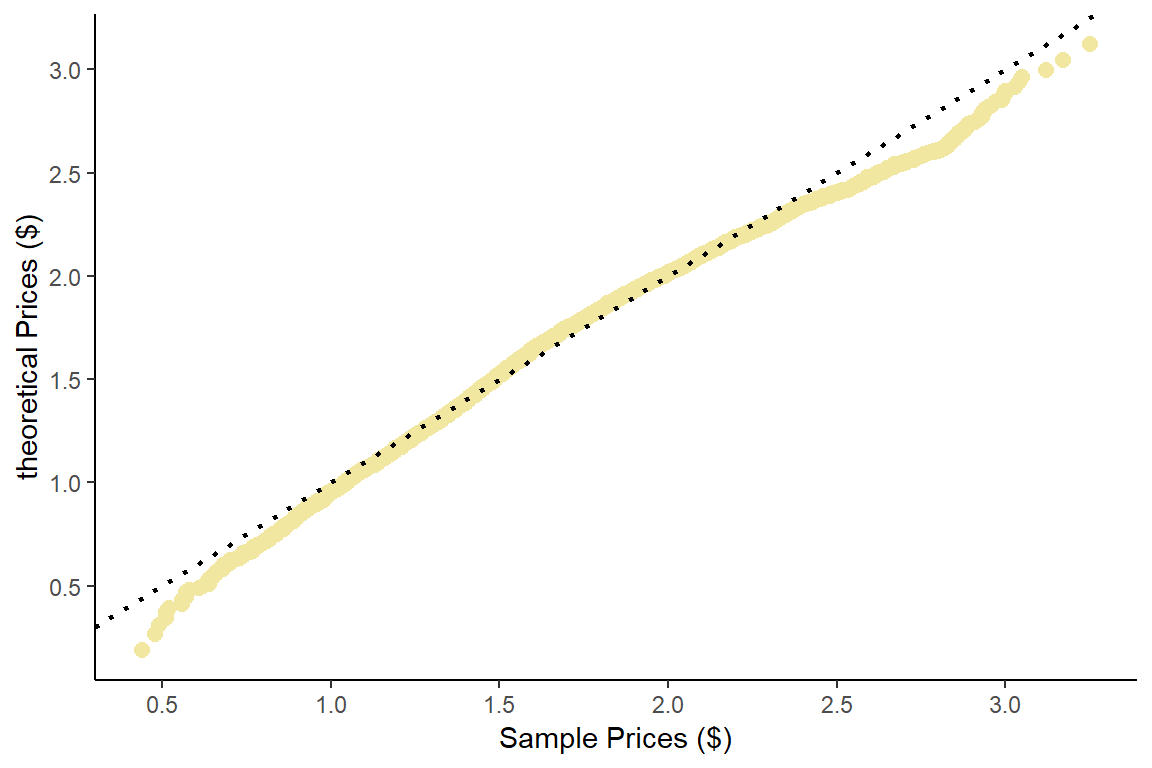This is an exceptionally well behaved sample. In fact, the histogram is clear enough that many analysts would not even bother to make the QQ plot for this sample. This is infrequently the case, however.

Let’s conclude by looking at one more version. Here I’ve changed the theoretical quantiles to come from a chi-squared distribution instead of a normal. We previously observed that the chi-squared(4) distribution has a single peak like our data. How well will the data fit that distribution?

This time I’ve used a little trick (standardization) to rescale the data.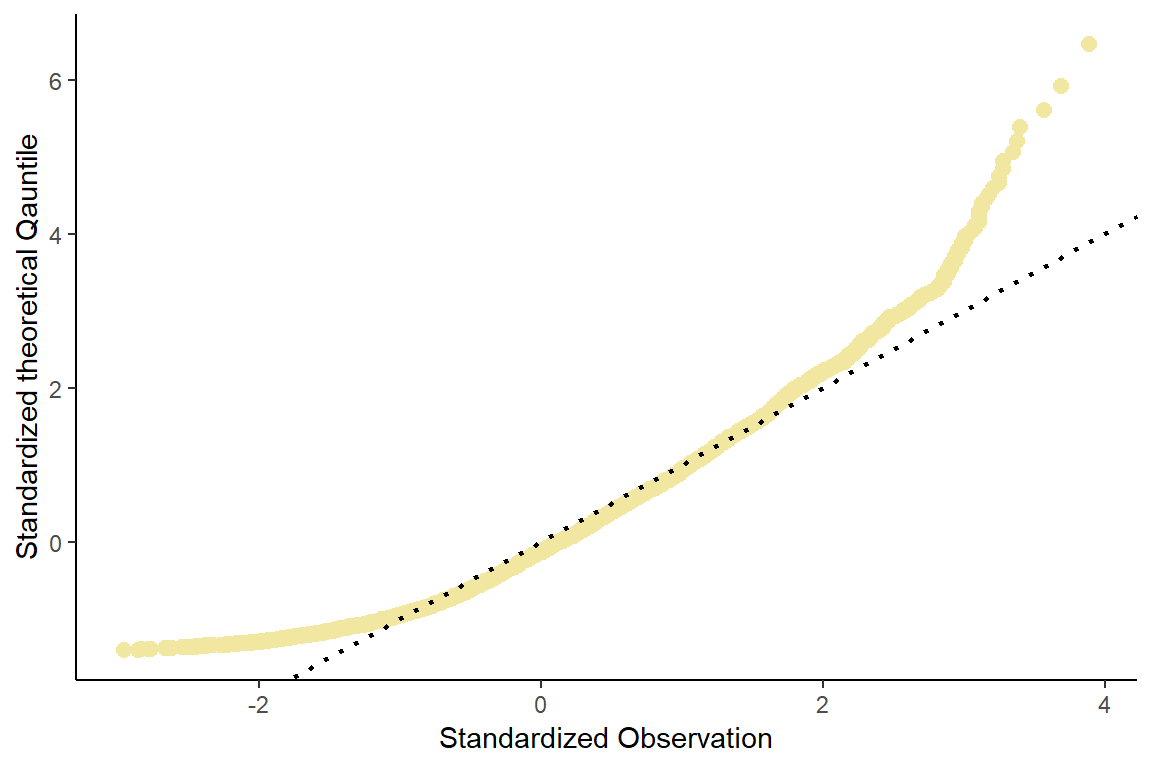The data fits this theoretical distribution much poorer than it did the normal distribution. The points do not form a straight line. The curve it forms is (unsurprisingly) similar to the QQ plot we created comparing the chi-squared (4) to the normal.

If we plot the histogram, we can see how the shape is much poorer: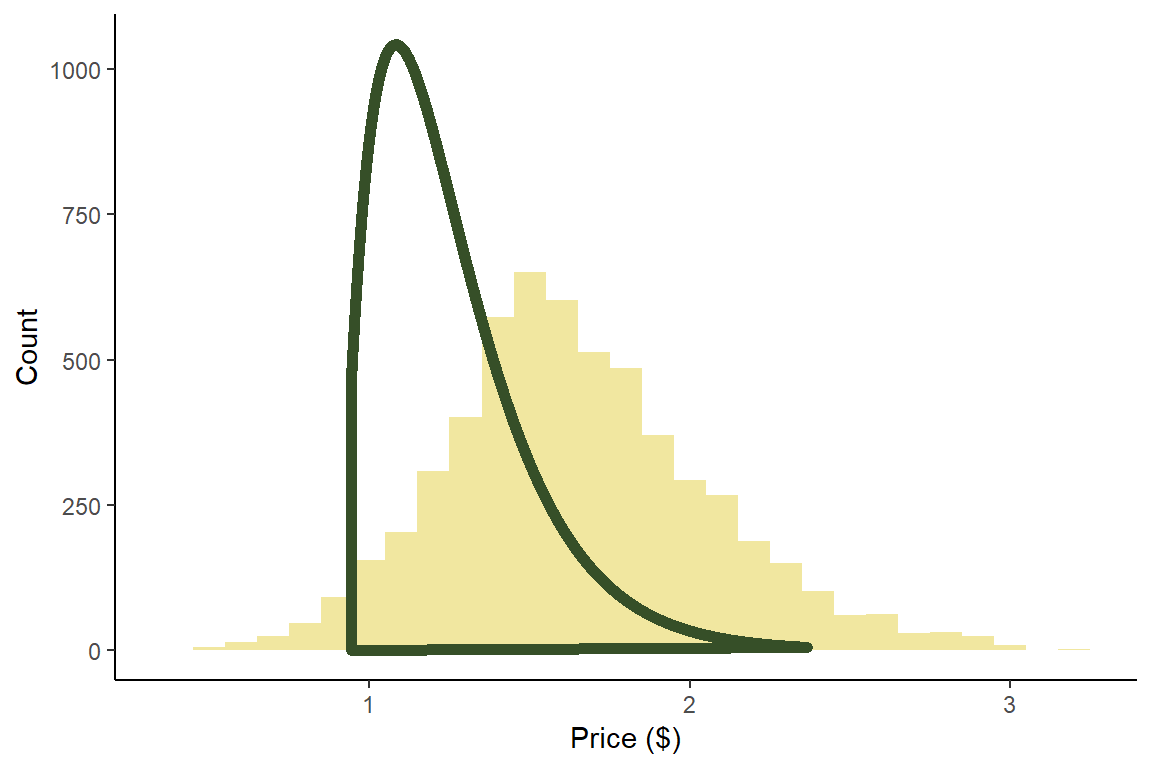The fact that the chi-squared distribution would have its quantiles more densely packed on the lower end of its distribution is reflected in the QQ plots slow increase on the left hand side of the graph.

### Data Audiation

It’s common wisdom that being able to visualize data is essential to understanding it but why should our eyes be the only sense we use?

During my process of thinking about distribution signatures I came up with a way that you can hear a distribution. This is meant to be a bit tongue in cheek but you’re still reading this so you probably like this stuff. Each signature’s sound begins with a bell and is played out with harsh beeps. Behold the sounds of distributions!

#### Sounds

##### Normal